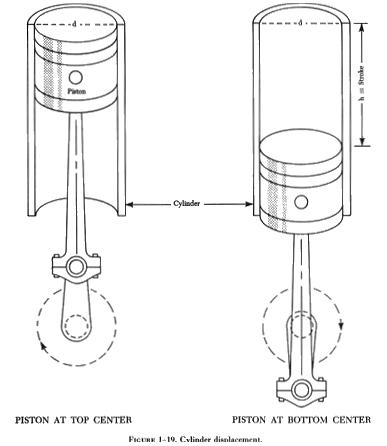COMPUTINGTHEVOLUMEOFSOLIDS COMPUTING THE VOLUME OF SOLIDS

Solids are objects with three dimensions - length, breadth, and thickness. They are of many shapes, the most common of which are prisms, cylinders, pyramids, cones, and spheres. Occasionally, it is necessary to determine the volume of a rectangle, a cube, a cylinder, or a sphere.

Since all volumes are not measured in the same units, it is necessary to know all the common units of volume and how they are related to each other. For example, the mechanic may know the volume of a tank in cubic feet or cubic inches, but when the tank is full of gasoline, he will be interested in how many gallons it contains. The following table shows the relationship between some of the common units of volume.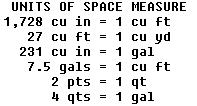Volume of a Rectangular Solid

 A rectangular solid is a solid bounded by rectangles. In other words, it is a square cornered volume such as a box (figure 1-16). If the solid has equal dimensions, it is called a cube. The formula for determining the volume of a rectangular solid may be expressed thus:         V = lwh    where: V = Volume.           l = length.           w = width.           h = height.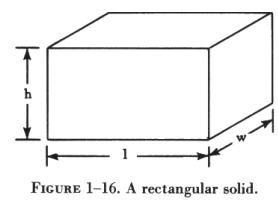EXAMPLE

A rectangular shaped baggage compartment measures 5 feet 6 inches in length, 3 feet 4 inches in width, and 2 feet 3 inches in height. How many cubic feet of baggage will it hold?

FIRST: Substitute the known values into the formula.

V = lwh

V = 5'6" x 3'4" x 2'3".

NEXT: Solve the formula for the unknown value.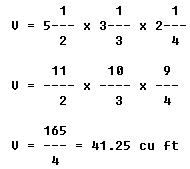If the rectangular solid is in the shape of a cube (figure 1-17), the formula can be expressed as the cube of the sides: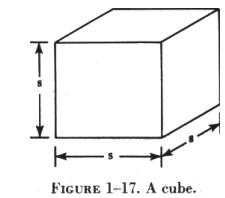V = S3 where V is the volume and S is the side measurement of the cube. Area and Volume of a Cylinder A solid having the shape of a can, length of pipe, or other such object is called a cylinder. The ends of a cylinder are identical circles as shown in figure 1-18.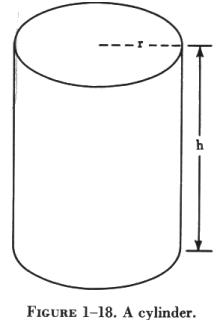Surface Area

The surface area of a cylinder is found by multiplying the circumference of the base by the altitude. The formula may be expressed as:

A =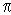x d x h

where A is the area, p is the given constant, d is the diameter, and h is the height of the cylinder.

EXAMPLE
How many square feet of aluminum sheet would be needed to fabricate a cylinder 12 feet long and 3 feet 6 inches in diameter?

FIRST: Substitute the known values in the formula.

A =x d x h

A = 3.1416 x 3'6" x 12'

NEXT: Solve the formula for the unknown value.

A = 3.1416 x 3.5' x 12'

A = 132.95, or 133 sq ft
Volume
The volume of a cylinder may be found by multiplying the cross-sectional area by the height of the cylinder. The formula may be expressed as:

V =x r2 x h

 where V is the volume; p is the given constant; r2 is the square of the radius of the cylinder; and h is the height of the cylinder (figure 1-19).    EXAMPLE      The cylinder of an aircraft engine has a bore (inside diameter) of 5.5 inches, and the engine has a stroke of 5.5 inches. What is the piston displacement of one cylinder? The stroke represents the height of the cylinder to be measured, because the volume displaced by the piston depends on the length of the stroke.      FIRST: Substitute the known values in the formula.         V =r2 h         V = (3.1416) (2.752) (5.5)      NEXT: Solve the formula for the unknown value.         V = 17.28 x 7.56         V = 130.64 cu in